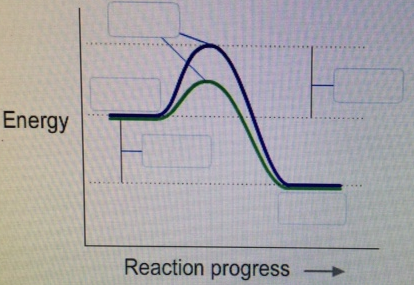# Problem: Label this diagram ΔE   reactants   products   Ea   transition stateWhich curve represents the catalyzed reaction? a) Blue(top) b) green (bottom)

###### FREE Expert Solution

Recall that an energy diagram is usually read from left to right.

The components of a one-step energy diagram are:

• Reactants: are placed on the left/beginning of the energy diagram

• Products: are placed on the right/end of the energy diagram

• Transition state: is the state with the highest energy in the energy diagram

• Energy change (ΔE or ΔG˚): is the difference in energy between products and reactants

• Activation energy (Ea): is the difference in energy between reactants and transition state

97% (490 ratings)###### Problem Details

Label this diagramΔE   reactants   products   Ea   transition state

Which curve represents the catalyzed reaction?

a) Blue(top)

b) green (bottom)

Frequently Asked Questions

What scientific concept do you need to know in order to solve this problem?

Our tutors have indicated that to solve this problem you will need to apply the Energy Diagram concept. If you need more Energy Diagram practice, you can also practice Energy Diagram practice problems.

What professor is this problem relevant for?

Based on our data, we think this problem is relevant for Professor Zawily's class at IOWA.